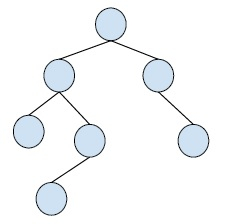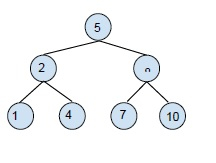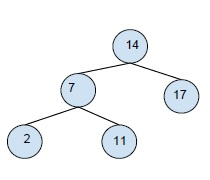# Binary Tree to Binary Search Tree Conversion in C++

A binary tree is a special type of tree in which each node of the tree can have at most two child nodes. These child nodes are known as the right child and left child.

A simple binary tree is −Binary search tree (BST) is a special type of tree which follows the following rules −

• left child node’s value is always less than the parent Note

• The right child node has a greater value than the parent node.

• all the nodes individually form a binary search tree.

Example of a binary search tree (BST)A binary search tree is created in order to reduce the complexity of operations like search, find minimum and maximum.

Here, we are given a binary tree and we have to convert this binary tree (BT) to a binary search tree (BST). In this conversion, the original structure of the binary tree should not be changed.

let's take an example to understand how to convert a BT into BST

InputOutputThis conversion of a binary tree to a binary search tree takes place using three steps. they are −

Step 1 − store data in order traversal of a binary tree into array arr[].

Step 2 − sort the array arr[] using any sorting technique.

Step 3 − Now, do the inorder traversal of the tree and e copy the elements of an array to the nodes of the tree one by one.

## Example

Live Demo

#include<stdio.h>
#include<stdlib.h>
struct node{
int data;
struct node *left;
struct node *right;
};
void Inordertraversal(struct node* node, int inorder[], int *index_ptr){
if (node == NULL)
return;
Inordertraversal(node->left, inorder, index_ptr);
inorder[*index_ptr] = node->data;
(*index_ptr)++;
Inordertraversal(node->right, inorder, index_ptr);
}
int countNodes(struct node* root){
if (root == NULL)
return 0;
return countNodes (root->left) +
countNodes (root->right) + 1;
}
int compare (const void * a, const void * b){
return( *(int*)a - *(int*)b );
}
void arrayToBST (int *arr, struct node* root, int *index_ptr){
if (root == NULL)
return;
arrayToBST (arr, root->left, index_ptr);
root->data = arr[*index_ptr];
(*index_ptr)++;
arrayToBST (arr, root->right, index_ptr);
}
struct node* newNode (int data){
struct node *temp = new struct node;
temp->data = data;
temp->left = NULL;
temp->right = NULL;
return temp;
}
void printInorder (struct node* node){
if (node == NULL)
return;
printInorder (node->left);
printf("%d ", node->data);
printInorder (node->right);
}
int main(){
struct node *root = NULL;
root = newNode(17);
root->left = newNode(14);
root->right = newNode(2);
root->left->left = newNode(11);
root->right->right = newNode(7);
printf("Inorder Traversal of the binary Tree: \n");
printInorder (root);
int n = countNodes(root);
int *arr = new int[n];
int i = 0;
Inordertraversal(root, arr, &i);
qsort(arr, n, sizeof(arr), compare);
i = 0;
arrayToBST (arr, root, &i);
delete [] arr;
printf("\nInorder Traversal of the converted BST: \n");
printInorder (root);
return 0;
}

## Output

Inorder Traversal of the binary Tree:
11 14 17 2 7
Inorder Traversal of the converted BST:
2 7 11 14 17